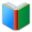# List 1 for 7th Grade MATH

Middle School Words: List 1 for 7th Grade MATH, all words of the list are in one page. You can download and print the worksheet from your browser directly.
 Free Online Vocabulary TestK12, SAT, GRE, IELTS, TOEFL
 Actions upon current list

 All lists of current grdae
Spelling Words:

Arts Words:

Social Words:

Math Words:

Science Words:

Literature Words:The Shoes of FortuneThe ShadowThe Adventures of Sherlock HolmesAdventures of Huckleberry Finn
 Worksheet - Middle School Words: List 1 for 7th Grade MATH

 Print: List 1 for 7th Grade MATH

Exam Word - https://www.examword.com/

Middle School Words: List 1 for 7th Grade MATH

 absolute value acute triangle bisector corresponding angles discount equilateral triangle experimental probability exponential notation exterior angles interest interior angles isosceles triangle negative obtuse triangle outcome permutations positive radical sign ratio regular polygon rhombus right triangle scale factor scalene triangle theoretical probability transversal unit rate vertical angle